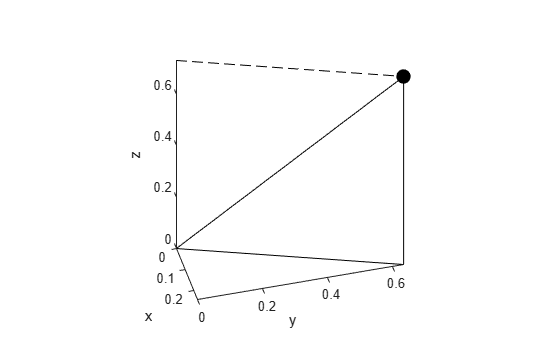# Plotting in Spherical Coordinate System

This example shows how to plot a point in spherical coordinates and its projection to Cartesian coordinates.

In spherical coordinates, the location of a point $P$ can be characterized by three coordinates:

• the radial distance $\rho$

• the azimuthal angle $\theta$

• the polar angle $\varphi$The relationship between the Cartesian coordinates $\left(x,y,z\right)$ of the point $P$ and its spherical coordinates $\left(\rho ,\theta ,\varphi \right)$ are:

`$\begin{array}{l}\mathit{x}=\rho \text{\hspace{0.17em}}\mathrm{sin}\text{\hspace{0.17em}}\varphi \text{\hspace{0.17em}}\mathrm{cos}\text{\hspace{0.17em}}\theta \text{\hspace{0.17em}}\\ \mathit{y}=\rho \text{\hspace{0.17em}}\mathrm{sin}\text{\hspace{0.17em}}\varphi \text{\hspace{0.17em}}\mathrm{sin}\text{\hspace{0.17em}}\theta \text{\hspace{0.17em}}\\ \mathit{z}=\rho \text{\hspace{0.17em}}\mathrm{cos}\text{\hspace{0.17em}}\varphi \end{array}$`

Plot the point $P$ using `plot3`. You can adjust the location of the point by changing the values of `rho`, `theta`, and `phi`.

```rho =0.8; theta =1.2; phi =0.75; x = rho*sin(phi)*cos(theta); y = rho*sin(phi)*sin(theta); z = rho*cos(phi); plot3(x,y,z,'ko','MarkerSize',10,'MarkerFaceColor','k') hold on```

Plot the line projection of the point $P$ onto the $z$-axis and the $xy$-plane using `fplot3`.

```syms r s xr = r*sin(phi)*cos(theta); yr = r*sin(phi)*sin(theta); zr = r*cos(phi); fplot3(xr,yr,zr,[0 rho],'k') fplot3(xr,yr,sym(0),[0 rho],'k') fplot3(xr,yr,sym(z),[0 rho],'k--') fplot3(sym(x),sym(y),rho*sin(s),[0 pi/2-phi],'k')```

Plot the planes that show the span of the azimuthal angle $\theta$ and the polar angle $\varphi$.

```syms s t xa = rho*sin(s)*cos(t); ya = rho*sin(s)*sin(t); fsurf(xa,ya,0,[0 phi 0 theta],'FaceColor','b','EdgeColor','none') syms u v xp = u*sin(v)*cos(theta); yp = u*sin(v)*sin(theta); zp = u*cos(v); fsurf(xp,yp,zp,[0 rho 0 phi],'FaceColor','g','EdgeColor','none') xlabel('x') ylabel('y') zlabel('z') view(115,30) axis equal; hold off```## Support

#### Mathematical Modeling with Symbolic Math Toolbox

Get examples and videos